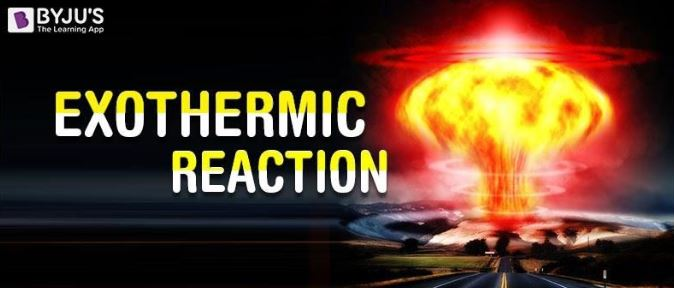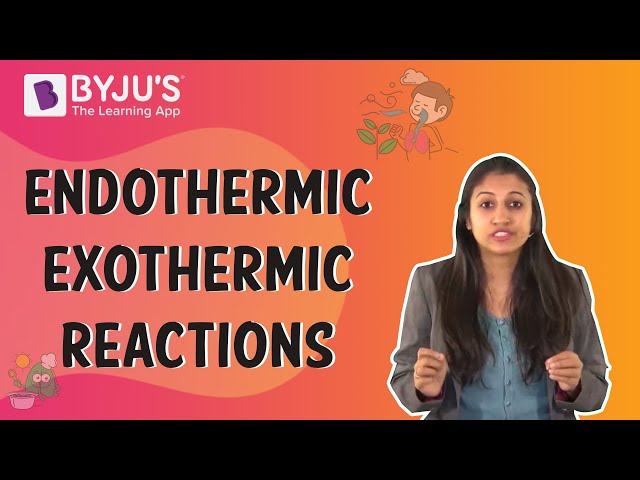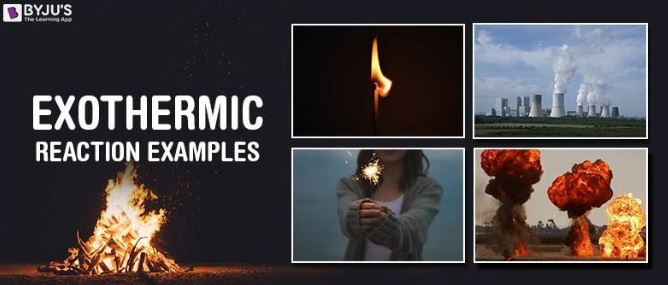Checkout JEE MAINS 2022 Question Paper Analysis : Checkout JEE MAINS 2022 Question Paper Analysis :

# Exothermic Reaction

An Exothermic reaction is a chemical reaction that involves the release of energy in the form of heat or light. These reactions are the opposite of endothermic reactions and can be expressed in a chemical equation as follows: Reactants → Products + Energy

## What is an Exothermic Reaction?

An exothermic reaction is a reaction in which energy is released in the form of light or heat. Thus in an exothermic reaction, energy is transferred into the surroundings rather than taking energy from the surroundings as in an endothermic reaction. In an exothermic reaction, the change in enthalpy ( ΔH) will be negative.Therefore, it can be understood that the net amount of energy required to initiate an exothermic reaction is less than the net amount of energy released by the reaction. When a calorimeter, a device used to measure the heat released by a chemical reaction, the net amount of heat energy that flows through the device is equal to the negative of the total energy change of the system.

However, it is extremely difficult to measure or even calculate the absolute total of energy in a given chemical system. Therefore, the energy change (or the enthalpy change, denoted by ΔH) is measured instead. The relation between the value of ΔH and the bond energies of the reaction can be given by the following equation.

ΔH = (energy used in the bond formation that yields products) – (energy released when the reactant bonds are broken)

Therefore, it can be understood that an exothermic reaction will always have a negative value for the change in enthalpy, i.e. ΔH < 0.

For the measurement of the enthalpy change of combustion reactions, bomb calorimeters are very suitable devices.

## Recommended Video## Examples of Exothermic Reactions

Exothermic reactions are everywhere, from the burning of a candle to the nuclear fusion reactions taking place in the sun. An image detailing some of the exothermic reactions which are used by human beings for beneficial purposes is provided below.Some common examples of exothermic reactions that are vital to the everyday lives of human beings are listed below.

### Combustion

• Combustion refers to the process where a substance burns in the presence of Oxygen, giving off heat and light in the process.
• These reactions give out good amounts of energy in the form of heat, but also form some byproducts such as smoke.
• An example of a combustion reaction would be the combustion of methane which can be represented as follows: CH4 + O2 → CO2 + H2O + Heat
• Another example of a combustion reaction is the combustion of glucose, given by the following reaction: C6H12O6 + 6O2 → 6CO2 + 6H2O + Energy

### Detonation of Nitroglycerin

• Nitroglycerin has strong explosive powers which can be used via its detonation.
• In the process of detonating nitroglycerin, the gases generated, at room temperature and pressure would occupy over 1,200 times the original volume.
• Nitroglycerin is one of the hottest detonating high explosives, detonating at temperatures up to 5000oC. Therefore, it is an exothermic reaction with very high ΔH values.

### Nuclear Fission of Uranium-235

• The nuclear fission of one atom of uranium-235 releases more than 2.5 million times the energy that is produced from the combustion of coal.
• The fission of one atom can cause a chain reaction in which the neutron from the fission of one uranium-235 atom can strike another atom, leading to its nuclear fission.
• This isotope of uranium is used in nuclear power plants, in which the fission reaction is controlled with the help of control rods that are capable of absorbing neutrons.

### Neutralization Reactions

• Neutralization reactions are chemical reactions wherein the reactants include an acid and a base, which go on to combine to yield a salt and water.
• These reactions neutralize the pH of the reacting species and hence are named after this neutralizing feature.
• The standard enthalpy change (ΔH) for the reaction between a proton (H+ ion) and a hydroxide ion (OH ion) is -57.30 kJ/mol. Therefore, neutralization reactions are considered to be exothermic reactions.

### Why is Respiration an Exothermic Reaction?

Respiration is the process by which humans take in oxygen and give out carbon dioxide. The chemical equation of this process can be represented as follows:

C6H12O6 + 6O2 → 6CO2 + 6H2O + Energy

In the reaction given above, it can be noted that C6H12O6 is the formula for glucose, which combines with the oxygen inhaled by humans to form carbon dioxide, water, and energy.

This energy produced in the reaction implies that the process of respiration is an exothermic reaction.

## Frequently Asked Questions – FAQs

### Define exothermic reaction.

An Exothermic reaction is a chemical reaction that involves the release of energy in the form of heat or light.

### What is a Combustion example?

Combustion refers to the process where a substance burns in the presence of Oxygen, giving off heat and light in the process.

### How do we use combustion?

Thermal energy derived from the combustion of either fossil fuels such as coal or oil or from renewable fuels such as firewood is extracted for a range of uses, such as cooking, power generation or industrial or domestic heating. Combustion is also the only process actually used to fuel rockets.

### What is the sign of enthalpy change for an exothermic reaction?

The change in enthalpy ( ΔH) for an exothermic reaction will be negative.

### Give examples of exothermic reactions.

Combustion reaction, Neutralization reaction, and Respiration.